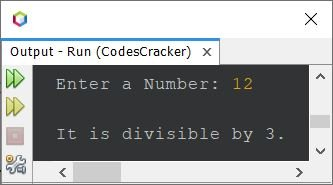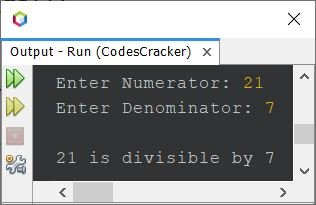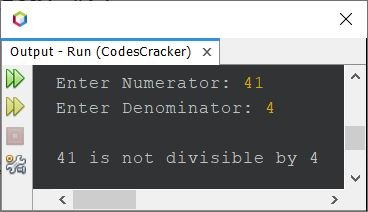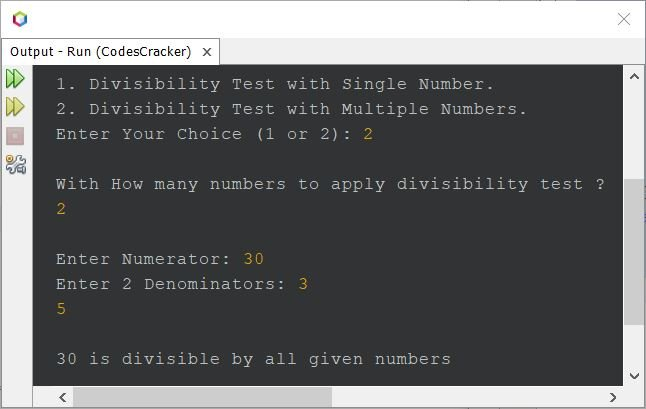# Java Program to Check if a Number is Divisible by Another Number

This article covers a program in Java to check if a number entered by user at run-time, is divisible by 3, 5, 7, 11 etc. or not. I've created multiple programs on the same topic. Let's start with very simple one.

## Check if a Number is Divisible by 3

The question is, write a Java program to check whether a number is divisible by 3 or not. The number must be received at run-time of the program. Here is its answer. I mean, the program given below is the answer to this question:

```import java.util.Scanner;

public class CodesCracker
{
public static void main(String[] args)
{
int num, den=3;
Scanner s = new Scanner(System.in);

System.out.print("Enter a Number: ");
num = s.nextInt();

if(num%den==0)
System.out.println("\nIt is divisible by 3.");
else
System.out.println("\nIt is not divisible by 3.");
}
}```

Here is its sample run with user input 12 as number to check whether it is divisible by 3 or not:Here is another sample run with user input 13:## Check if a Number is Divisible by 5

To create a program that can check whether a number entered by user is divisible by 5 or not, just replace 3 with 5 from above program. Rest of all the codes are same.

## Check if a Number is Divisible by Another Number

This is the main program that we need. Because using this program, we can test divisibility with any combination of numbers. For example, we can check whether a number is divisible by 11 or not, whether a number is divisible by 7 or not and so on.

```import java.util.Scanner;

public class CodesCracker
{
public static void main(String[] args)
{
int num, den;
Scanner s = new Scanner(System.in);

System.out.print("Enter Numerator: ");
num = s.nextInt();
System.out.print("Enter Denominator: ");
den = s.nextInt();

if(num%den==0)
System.out.println("\n" +num+" is divisible by " +den);
else
System.out.println("\n" +num+" is not divisible by " +den);
}
}```

Here is its sample run with user input 21 as numerator and 7 as denominator to check whether the number 21 is divisible by 7 or not:Here is another sample run with user input 41 as numerator and 4 as denominator to check whether the number 41 is divisible by 4 or not:Now let's modify the above program to provide user, two options to go with. One to check if a number is divisible by another single number, and other to check whether a number is divisible by multiple numbers or not:

```import java.util.Scanner;

public class CodesCracker
{
public static void main(String[] args)
{
int num, choice;
Scanner s = new Scanner(System.in);

System.out.println("1. Divisibility Test with Single Number.");
System.out.println("2. Divisibility Test with Multiple Numbers.");
System.out.print("Enter Your Choice (1 or 2): ");
choice = s.nextInt();
if(choice==1)
{
int den;
System.out.print("\nEnter Numerator: ");
num = s.nextInt();
System.out.print("Enter Denominator: ");
den = s.nextInt();
if(num%den==0)
System.out.println("\n" +num+" is divisible by " +den);
else
System.out.println("\n" +num+" is not divisible by " +den);
}
else if(choice==2)
{
int total, count=0;
System.out.println("\nWith How many numbers to apply divisibility test ? ");
total = s.nextInt();
int[] den = new int[total];

System.out.print("\nEnter Numerator: ");
num = s.nextInt();
System.out.print("Enter " +total+ " Denominators: ");
for(int i=0; i<den.length; i++)
{
den[i] = s.nextInt();
if(num%den[i]!=0)
count=1;
}

if(count==0)
System.out.println("\n" +num+" is divisible by all given numbers");
else
System.out.println("\n" +num+" is not divisible by all given numbers");
}
else
System.out.println("\nInvalid Choice!");
}
}```

Here is its sample run with user input 2 as choice, 2 as total numbers, 30 as numerator, 3 and 5 as two numbers to check whether 30 is divisible by 3 and 5 or not:Java Online Test

« Previous Program Next Program »